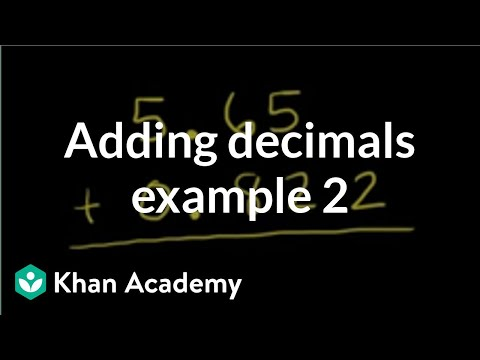Video

# One-step addition & subtraction equations: fractions & decimals (Full video)

Khan Academy

## Supporting grades: 6

Description: Learn how to solve one-step addition and subtraction equations that have fractions and decimals in them. Let's give ourselves some practice solving equations. So let's say we had the equation 1/3 plus A is equal to 5/3. So there's a bunch of different ways of doing this, and this is one of the fun things about equations is there's no exactly one right way to do it.

You must log inorsign upif you want to:*

*Teacher Advisor is 100% free.

### Other videos you might be interested in### Using Substitution to Understand Equations and Inequalities (Full video)

#### Student Achievement Partners